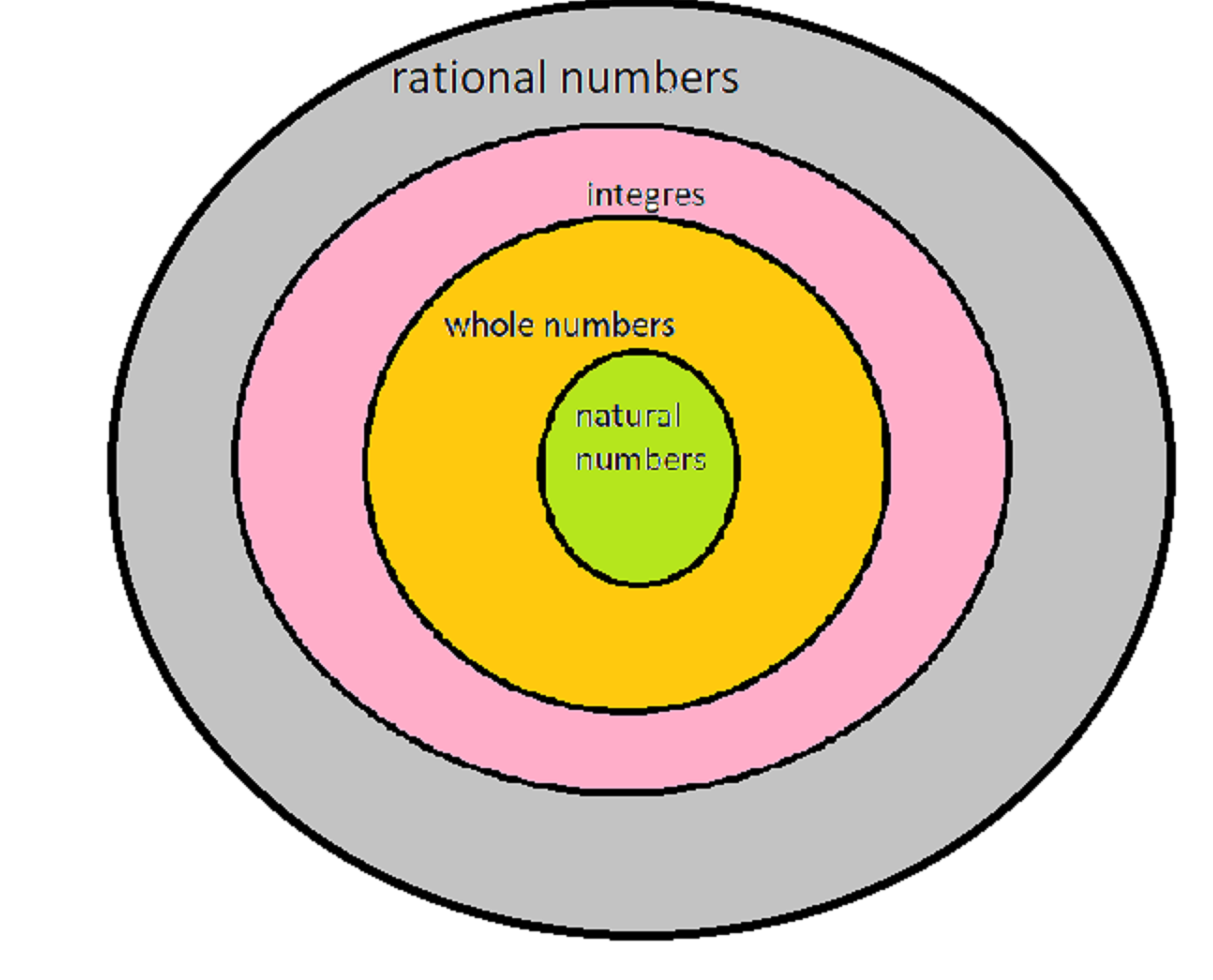October 1, 2023

Contents

# Various types of numbers: natural numbers, whole numbers, integers and rational numbers

## Various types of numbers :

According to the properties and how they are represented in the number line, there are various types of numbers . The numbers are classified into different types, like natural number, integers,  whole numbers   etc.

# Natural numbers

The natural numbers are those numbers that are used for counting and ordering.  The set of natural numbers is often denoted by the symbol ,

The least natural number is 1 and there are infinitely  many natural numbers.  They are located  at the right side of the number line (after 0)

Properties of natural numbers:

(i) Closure property: The sum  and  multiplication of any two natural numbers is always a natural number. This is called “Closure property of  addition and multiplication”  of natural numbers. Thus, is closed under addition and multiplication .  If a and b are any two natural numbers, then and is also a natural number. e.g. 7+2=9    and  is also a natural number.

The difference between any two natural numbers need not be a natural number.

Example :    3 – 5  =  -2 is a not natural number. Hence   is not closed under subtraction.

Similarly   is also not closed under division.

(ii) Commutative property :  Addition  and multiplication of two natural numbers is   commutative. If and are any two natural numbers, then,   and

Subtraction  and division of two natural numbers is not commutative.

If a and b are any two natural numbers,  then     and   $a÷b$$b÷a$.

e.g.  (i) 5 – 3  =  2 and  3 – 5  =  -2 .  Hence    5 – 3  ≠  3 – 5

(ii)2 ÷ 1  =  2 and 1 ÷ 2  =  1.5 .  Hence    2 ÷ 1  ≠  1 ÷ 2

Therefore, Commutative property is not true for subtraction and addition.

(iii) Associative property : Addition  and multiplication of natural numbers is associative.

If a, b and c  are any three natural numbers,  then    and .

e.g.   (a)      and  .    Hence,
(b)    and  . Hence

Subtraction  and division of natural numbers  is not associative .

It means for any natural number a , b and c      and $a÷\left(b÷c\right)$$\left(a÷b\right)÷c$

(iv)Identity element :  The additive identity  of a natural numbers  is zero and multiplicative identity of natrural numbers is 1.  If a is any natural number, then    and

(v)Distributive Property:    Multiplication of natural numbers is distributive over addition and subtraction . If a, b and c  are any three natural numbers,  then a x (b + c)  =  ab + ac and a x (b – c)  =  ab – ac.

## Whole numbers

All natural numbers together with  ‘0’  are called  whole numbers.  The set of Whole numbers is denoted by W and written as W={0,1,2,3,4,5,……………………}

### Integers

It includes  all natural  numbers , 0  and negative of natural  numbers . It is denoted by ,representation of integers on number line

Negative  integers are on the left side of 0

Positive integers are on the right side of the zero

0 is neither +ve  nor -ve.

#### Rational numbers

The numbers of the form where and are integers  and , are known as rational numbers. The collection of rational numbers is denoted  by and is written as

Thus,   , etc. are all rational numbers.

Rational numbers include natural numbers , whole numbers and integers since every natural numbers , whole numbers and integers can be written as

where   is either any natual no. or  whole number or integer.You might also be interested in:#### Bina singh

View all posts by Bina singh →
ssc chsl 2023 tier 1 cut off NIRF Rankings 2023 : Top 10 Engineering colleges in India CBSE Compartment Exam 2023 Application Form SSC CGL 2023 Notification: Important Dates UPSC Recruitment 2023